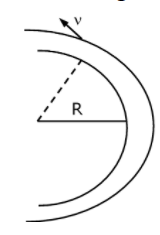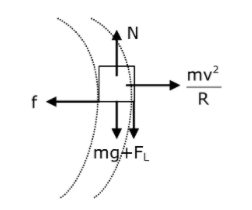# A modern grand-prix racing car of mass m

A modern grand-prix racing car of mass m is travelling on a flat track in a circular arc of radius R with a speed v.If the coefficient of static friction between the tyres and the track is μs, then the magnitude of negative lift F1acting downwards on the car is : (Assume forces on the four tyres are identical and g = acceleration due to gravity)a. $$m(\frac{v^{2}}{\mu _{s}R}-g)$$

b. $$m(\frac{v^{2}}{\mu _{s}R}+g)$$

c. $$m(g-\frac{v^{2}}{\mu _{s}R})$$

d. $$- m(g+\frac{v^{2}}{\mu _{s}R})$$Static friction = f =mv2/R from force balance along radial direction,

Normal reaction = N =mv2/Rfrom force balance along vertical,

μ(mg + FL) = mv2/R

mg + FL = mv2/Rμ

FL = (mv2/μR) – mg

= m(v2/μR – g)(3)(0)